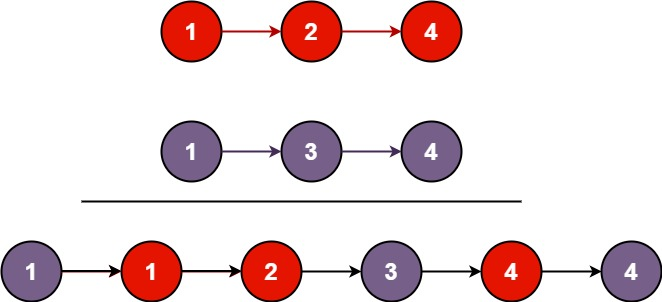### LeetCode• ㊗️
• 大家
• offer
• 多多！

## Problem

You are given the heads of two sorted linked lists list1 and list2.

Merge the two lists in a one sorted list. The list should be made by splicing together the nodes of the first two lists.

Example 1:Input: list1 = [1,2,4], list2 = [1,3,4]
Output: [1,1,2,3,4,4]


Example 2:

Input: list1 = [], list2 = []
Output: []


Example 3:

Input: list1 = [], list2 = 
Output: 


Constraints:

• The number of nodes in both lists is in the range [0, 50].
• -100 <= Node.val <= 100
• Both list1 and list2 are sorted in non-decreasing order.

## Code

class Solution {
public ListNode mergeTwoLists(ListNode l1, ListNode l2) {
ListNode dummy = new ListNode(-1);
ListNode curr = dummy;

while(l1 != null || l2 != null){
int x = l1 == null ? Integer.MAX_VALUE : l1.val;
int y = l2 == null ? Integer.MAX_VALUE : l2.val;

if(x < y){
curr.next = new ListNode(x);
l1 = l1.next;
} else {
curr.next = new ListNode(y);
l2 = l2.next;
}

curr = curr.next;
}

return dummy.next;
}
}

class Solution {
public ListNode mergeTwoLists(ListNode l1, ListNode l2) {
ListNode dummy = new ListNode(-1);
ListNode curr = dummy;

while(l1 != null && l2 != null){
if(l1.val < l2.val) {
curr.next = l1;
l1 = l1.next;
} else {
curr.next = l2;
l2 = l2.next;
}

curr = curr.next;
}

if(l1 != null) {
curr.next = l1;
}

if(l2 != null) {
curr.next = l2;
}

return dummy.next;
}
}